So the other day, I solved this curious mathematics puzzle using repeated applications of Pythagoras’s theorem and a little bit of algebra.

Now I realize that there is a much simpler form of the proof.

The exercise was to prove that, given two semicircles drawn into a bigger circle as shown below, the sum of the areas of the semicircles is exactly half that of the larger circle.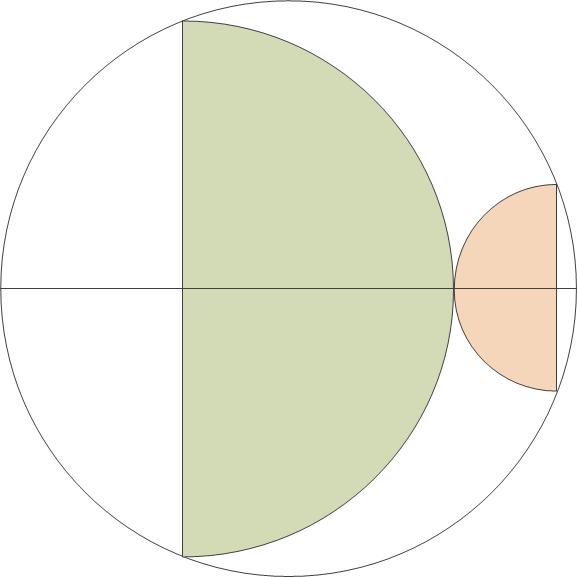Again, I’m inserting a few blank lines before presenting my proof.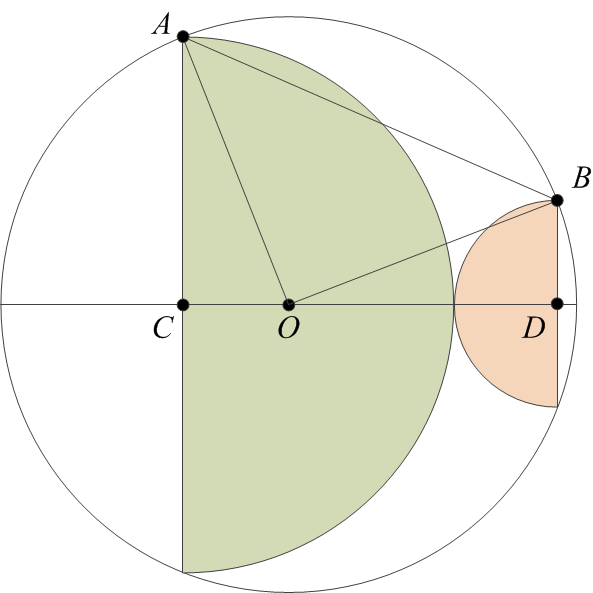Once again I am labeling some vertices in the diagram for easy reference.

Our goal is to prove that the area of a circle with radius AO is twice the sum of the areas of two semicircles, with radii AC and BD. But that is the same as proving that the area of a circle with radius AO is equal to the sum of the areas of two circles, with radii AC and BD.

The ACO< angle is a right angle. Therefore, the area of a circle with radius AO is the sum of the areas of circles with radii AC and CO. (To see this, just multiply the theorem of Pythagoras by π.) So if only we could prove that CO = BD, our proof would be complete.

Since AO = BO, they are the sides of the isosceles triangle ABO. Now if we were to pick a point O on the line CD such that CO‘ = BD, the ACO and ODB triangles will be identical (CD being the sum of AC and BD by construction). Therefore, AO‘ = BO, and the ABO triangle would be another isosceles triangle with its third vertex on the CD line. Clearly that is not possible, so O = O, and therefore, CO = BD. This concludes the proof.

The other day, I ran across a cute geometry puzzle on John Baez’s Google+ page. I was able to solve it in a few minutes, before I read the full post that suggested that this was, after all, a harder-than-usual area puzzle. Glad to see that, even though the last high school mathematics competition in which I participated was something like 35 years ago, I have not yet lost the skill.

Anyhow, the puzzle is this: prove that the area of the two semicircles below is exactly half the area of the full circle.I am going to insert a few blank lines here before providing my solution.

I start with labeling some vertices on the diagram and also drawing a few radii and other lines to help.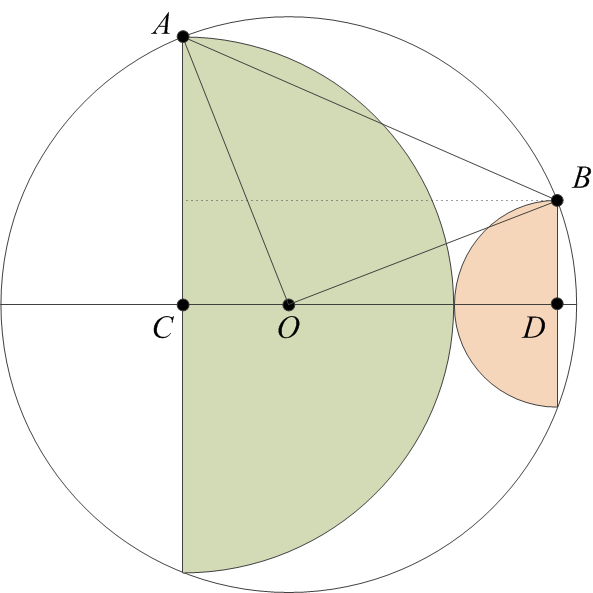Next, let’s call the radii of the two semicircles as $$a$$ and $$b$$. Then, we have
\begin{align}
(AC)&= a,\\
(BD)&= b.
\end{align}Now observe that
\begin{align}
(OA) = (OB) = r,
\end{align}and also
\begin{align}
(CD)&= a + b,\\
(OD)&= a + b~- (OC).
\end{align}The rest is just repeated application of the theorem of Pythagoras:
\begin{align}
(OC)^2&= r^2 – a^2,\\
(OD)^2&= r^2 – b^2,
\end{align}followed by a bit of trivial algebra:
\begin{align}
(OC)^2 + a^2&= [a + b – (OC)]^2 + b^2,\\
0&= 2(a + b)[b – (OC)],\\
(OC)&= b.
\end{align}Therefore,
\begin{align}
a^2+b^2=r^2,
\end{align}which means that the area of the full circle is twice the sum of the areas of the two semicircles, which is what we set out to prove.

I guess I have not yet lost my passion for pointless, self-serving mathematics.

Our cat Szürke is not only still with us, but his condition continues to improve. This is no small Christmas miracle. Made possible, well, by modern veterinary science and the money spent to pay for it, but also by the caring of veterinarians and their staff at Beechwood Animal Hospital (our “local” vet) and Alta Vista Animal Hospital (where Szürke received numerous transfusions and emergency care.)Year after year, as Christmas Eve nears, I recall the Christmas message of Apollo 8 astronaut Frank Borman. Here is what he said in 1968, 45 years ago: “And from the crew of Apollo 8, we close with good night, good luck, a Merry Christmas and God bless all of you – all of you on the good Earth.

Amen.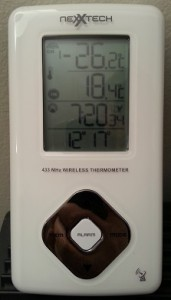Damn it’s cold this morning. Negative 26 Centigrade. Or 27 if I believe the local news. And it’s not even winter yet!

To be sure, I still prefer to live in the Great White North instead of any of the numerous southerly climates full of crazy people, but sometimes, it’s a bit too much. Like, when you feel like you need to put on a spacesuit just to step outside to grab your newspaper from your doorstep.

Yes, I still subscribe to a newspaper. Or rather, I again subscribe to a paper after canceling my Globe and Mail subscription more than a decade ago. I accepted their offer for a free three-month subscription back in the summer, and I became used to it. More importantly, I realized that there are things I’d never even read or hear about had they not been in the paper. Electronic media is great, but it tends to deliver the news that you actually want to hear. Especially as services like Google News or Facebook employ sophisticated algorithms that try to predict what you’re most likely to read based on your past behavior. So if you wish to step outside of your comfort zone, to have your views challenged, not simply confirmed… well, a newspaper helps.

Besides… as a last resort, you can also use a newspaper to start a fire to keep warm.

I am reading a very interesting paper by Christian Beck, recently published in Physical Review Letters.

Beck revives the proposal that at least some of the as yet unobserved dark matter in the universe may be in the form of axions. But he goes further: he suggests that a decade-old experiment with superconducting Josephson-junctions that indicated the presence of a small, unexplained signal may in fact have been a de facto measurement of the axion background in our local galactic neighborhood.

If true, Beck’s suggestion has profound significance: not only would dark matter be observable, but it can be observed with ease, using a tabletop experiment!

What is an axion? The Standard Model of particle physics (for a very good comprehensive review, I recommend The Standard Model: A Primer by Cliff Burgess and Guy Moore, Cambridge University Press, 2007) can be thought of as the most general theory based on the observed particle content in the universe. By “most general”, I mean specifically that the Standard Model can be written in the form of a Lagrangian density, and all the terms that can be present do, in fact, correspond to physically observable phenomena.

All terms except one, that is. The term, which formally reads

\begin{align}{\cal L}_\Theta=\Theta_3\frac{g_3^2}{64\pi^2}\epsilon^{\mu\nu\lambda\beta}G^\alpha_{\mu\nu}G_{\alpha\lambda\beta},\end{align}

where $$G$$ represents gluon fields and $$g_3$$ is the strong coupling constant ($$\epsilon^{\mu\nu\lambda\beta}$$ is the fully antisymmetric Levi-Civita pseudotensor), does not correspond to any known physical process. This term would be meaningless in classical physics, on account of the fact that the coupling constant $$\Theta_3$$ multiplies a total derivative. In QCD, however, the term still has physical significance. Moreover, the term actually violates charge-parity (CP) symmetry.

The fact that no such effects are observed implies that $$\Theta_3$$ is either 0 or at least, very small. Now why would $$\Theta_3$$ be very small? There is no natural explanation.

However, one can consider introducing a new scalar field into the theory, with specific properties. In particular this scalar field, which is called the axion and usually denoted by $$a$$, causes $$\Theta_3$$ to be replaced with $$\Theta_{3,{\rm eff}}=\Theta_3 + \left<a\right>/f_a$$, where $$f_a$$ is some energy scale. If the scalar field were massless, the theory would demand $$\left<a\right>/f_a$$ to be exactly $$-\Theta_3$$. However, if the scalar field is massive, a small residual value for $$\Theta_{3,{\rm eff}}$$ remains.

As for the Josephson-junction, it is a superconducting device in which two superconducting layers are separated by an isolation layer (which can be a normal conductor, a semiconductor, or even an insulator). As a voltage is introduced across a Josephson-junction, a current can be measured. The peculiar property of a Josephson-junction is the current does not vanish even as the voltage is reduced to zero: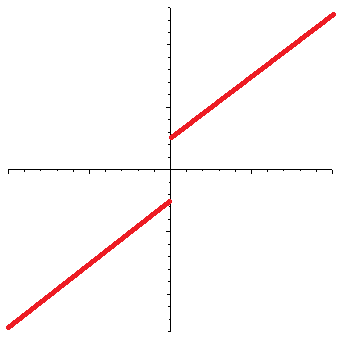(The horizontal axis is voltage, the vertical axis is the current. In a normal resistor, the current-voltage curve would be a straight line that goes through the origin.) This is the DC Josephson effect; a similar effect arises when an AC voltage is applied, but in that case, the curve is even more interesting, with a step function appearance.

The phase difference $$\delta$$ between the superconductors a Josephson-junction is characterized by the equation

\begin{align}\ddot{\delta}+\frac{1}{RC}\dot{\delta}+\frac{2eI_c}{\hbar C}\sin\delta&=\frac{2e}{\hbar C}I,\end{align}

where $$R$$ and $$C$$ are the resistance and capacitance of the junction, $$I_c$$ is the critical current that characterizes the junction, and $$I$$ is the current. (Here, $$e$$ is the electron’s charge and $$\hbar$$ is the reduced Planck constant.)

Given an axion field, represented by $$\theta=a/f_a$$, in the presence of strong electric ($${\bf E}$$) and magnetic ($${\bf B}$$) fields, the axion field satisfies the equation

\begin{align}\ddot{\theta}+\Gamma\dot{\theta}+\frac{m_a^2c^4}{\hbar^2}\sin\theta=-\frac{g_\lambda c^3e^2}{4\pi^2f_a^2}{\bf E}{\bf B},\end{align}

where $$\Gamma$$ is a damping parameter and $$g_\lambda$$ is a coupling constant, while $$m_a$$ is the axion mass and of course $$c$$ is the speed of light.

The formal similarity between these two equations is striking. Now Beck suggests that the similarity is more than formal: that in fact, under the right circumstances, the axion field and a Josephson-junction can form a coupled system, in which resonance effects might be observed. The reason Beck gives is that the axion field causes a small CP symmetry perturbation in the Josephson-junction, to which the junction reacts with a small response in $$\delta$$.

Indeed, Beck claims that this effect was, in fact, observed already, in a 2004 experiment by Hoffman, et al., who attempted to measure the noise in a certain type of Josephson-junction. In their experiment, a small, persistent peak appeared at a voltage of approximately 0.055 mV: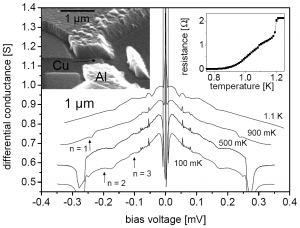If Beck is correct, this observation corresponds to an axion with a mass of 0.11 meV (that is to say, the electron is some five billion times heavier than this axion) and the local density of the axion field would be about one sixth the presumed dark matter density in this region of the Galaxy.

I don’t know if Beck is right or not, but unlike most other papers about purported dark matter discoveries, this one does not feel like clutching at straws. It passes the “smell test”. I’d be very disappointed if it proved to be true (I am not very much in favor of the dark matter proposal) but if it is true, I think it qualifies as a Nobel-worthy discovery. It is also eerily similar to the original discovery of the cosmic microwave background: it was first observed by physicists who were not at all interested in cosmology but instead, were just trying to build a low-noise microwave antenna.

Here is something new: America’s ever watchful National Security Agency is not content with spying in all the real lands of the world. Their interests also extend to imaginary realms, like the virtual world of Second Life and World of Warcraft.

Ostensibly, their concern is that terrorists around the world might be using online games for secret communication. The idea is not, in fact, new; for what it’s worth, a similar idea exists as a plot device in Margaret Atwood’s superb, dystopian Oryx and Crake trilogy.

So I guess I should count it as a blessing that other aspects of Atwood’s nightmarish future have not become reality yet. Instead of corporatist anarchy, all we have is a benevolent superstate ever more keen on enforcing Pax Americana. And who knows… our freedoms and privacy may be somewhat curtailed in this New World Order, but if the Roman example is any guide, it may be a small price to pay for centuries of stable prosperity.

Anyhow, for what it’s worth, as far as I know there is no spying going on in MUD1/British Legends and MUD2. I can actually vouch for MUD1 personally; I, after all, wrote the code for the current implementation.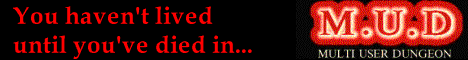Imagine a country in which small children are given coloring books figuring a leading politician.

Coloring books that describe the politician in “non-partisan and fact-driven” terms. A lengthy speech becomes a “magnificent feat”, during which the dear leader spoke with “clairvoyant precision”. The goals of political opponents are “worse than any war”.

This coloring book is “approved by teachers and educators”. It is “designed to be a fun, educational tool”. Parents are encouraged to “Tell the truth – Tell it often – Tell the children”.

If you thought I was describing a North Korean coloring book featuring the “Great Successor” King Jong-un or his daddy or granddaddy, think again.

That is because the abomination that I just described was in fact published in the great United States of America. Its title: “Ted Cruz to the Future™ – Comic Coloring Activity Book“, published by Really Big Coloring Books®, Inc.

And it is available at Amazon for the bargain price of \$5.69. Or it was, anyway; presently, it is shown as “Temporarily out of stock.”

Here are two kittycats, Kifli (left) and Szürke. Szürke is the cat who caused us many a sleepless night in the past two months, with his mysterious anaemia.The good news is that he is holding steady, now nearly three weeks since his last transfusion. His red blood cell count is still not recovering the way it should, but we may have arrested the loss. My fingers remain firmly crossed.

Hard to believe but back in his heyday, Szürke was significantly heavier than his brother.

American news channels are abuzz with news about the revamped Obamacare Web site, healthcare.gov.

Moments ago, out of curiosity, I visited the site. To be precise, I wanted to search for news about healthcare.gov, so clicking on a link that actually took me to the site is something I did more by accident than by design.

Indeed, I only realized that I actually visited the site (and not just a news site page about the site) when I encountered the following error:Ah, the irony.